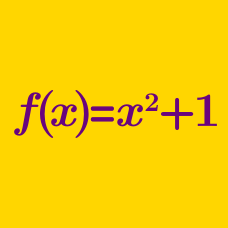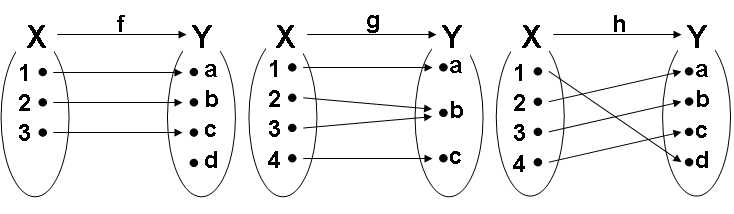Algebra

# Inverse FunctionsConsider 3 functions $f(x), g(x),$ and $h(x)$ above.

Which has an inverse function ?

Let $f(x)=2x-7,$ and let $g(x)=f^{-1}(x)$. What is the value of $g(15)+g^{-1}(17)$?

 Details and assumptions

• $g^{-1}(x)$ denotes the inverse function of $g(x)$.

If $f^{-1}(2)=0$ and $(f \circ f)(0) = 20$ for $f(x)=ax+b$, what is $a+b$?

Consider the function $f(x) =$ $\begin{cases} 2x & (x \ge 1) \\ 2(1-k)x + 2k & (x < 1). \end{cases}$

If $f^{-1}(x)$ exists, find the value of the constant $k.$

 Details and assumptions

• $f^{-1}(x)$ denotes the inverse function of $f(x).$

Consider a function $f: X \to Y,$ where $X = \{x |\, 0 \le x \le 1\}$ and $Y = \{y|\, a \le y \le b\}.$

If $f(x) = 3x + 2,$ and $f^{-1}(x)$ exists, find the values of $a , b.$

 Details and assumptions

• $f^{-1}(x)$ denotes the inverse function of $f(x).$
×